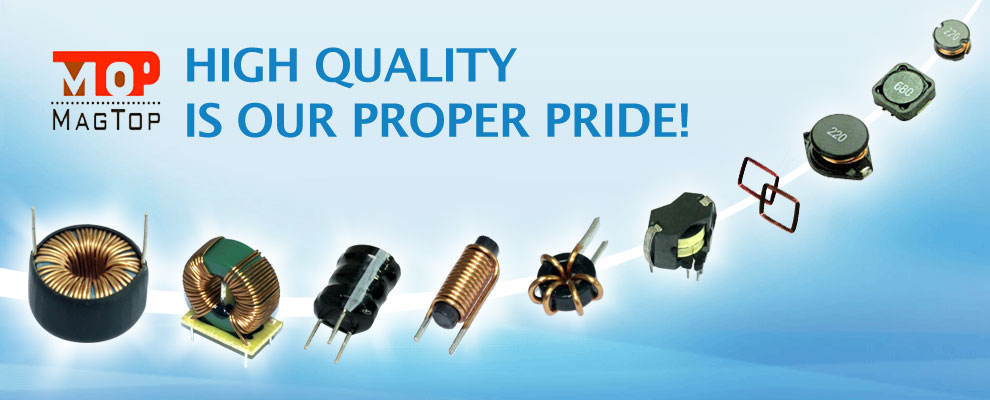# Basic knowledge of Inductor Choke Coils

##### author: MagTop Technology Department
20/12/2021
Inductance coils are made of wires wound around an insulating tube one by one. The wires are insulated from each other. The insulating tube can be hollow, or it can contain iron cores or magnetic powder cores, referred to as inductors. Expressed by L, the units are Henry (H), Milli Henry (mH), Micro Henry (uH), 1H=10^3mH=10^6uH.A. Classification of inductance
Classified according to the form of inductance: fixed inductance, variable inductance.
According to the nature of the magnetic conductor: air core coil, ferrite coil, iron core coil, copper core coil.
Classified by work nature: antenna coil, oscillating coil, choke coil, trap coil, deflection coil.
Classified by winding structure: single-layer coil, multi-layer coil, honeycomb coil.

B. the main characteristic parameters of the inductance coil
1. Inductance L
The inductance L represents the inherent characteristics of the coil itself and has nothing to do with the magnitude of the current. Except for special inductance coils (color code inductance), the inductance is generally not specifically marked on the coil, but marked with a specific name.
2. Inductive resistance XL
The hindering effect of the inductance coil on the alternating current is called the inductive reactance XL, and the unit is ohm. Its relationship with inductance L and alternating current frequency f is XL=2πfL.
3. Quality factor Q
The quality factor Q is a physical quantity that represents the quality of the coil, and Q is the ratio of the inductive reactance XL to its equivalent resistance, namely: Q=XL/R. The higher the Q value of the coil, the smaller the loss of the loop. The Q value of the coil is related to the DC resistance of the wire, the dielectric loss of the frame, the loss caused by the shield or the iron core, and the influence of the high-frequency skin effect. The Q value of the coil is usually tens to hundreds.
4. Distributed capacitance
The capacitance between the turns of the coil, between the coil and the shield, and between the coil and the base plate is called distributed capacitance. The existence of distributed capacitance reduces the Q value of the coil and the stability becomes worse. Therefore, the smaller the distributed capacitance of the coil, the better.

C. Commonly used coils
1. Single layer coil
Single-layer coils are wound around a paper tube or bakelite frame with insulated wires. Such as the medium wave antenna coil of a transistor radio.
2. Honeycomb coil
If the plane of the coil being wound is not parallel to the rotating surface, but intersects at a certain angle, this kind of coil is called a honeycomb coil. The number of times the wire is bent back and forth after one rotation is often called the number of bending points. The advantages of the honeycomb winding method are small size, small distributed capacitance, and large inductance. The honeycomb coils are all wound by the honeycomb winding machine, the more the turning points, the smaller the distributed capacitance
3. Ferrite core and iron powder core coil
The inductance of the coil is related to the presence or absence of a magnetic core. Inserting the ferrite core in the air-cored coil can increase the inductance and improve the quality factor of the coil.
4. Copper core coil
Copper core coils are widely used in the ultrashort wave range. The position of the copper core in the coil is used to change the inductance. This adjustment is more convenient and durable.
5. Color code inductor
The color code inductor is an inductor with a fixed inductance, and its inductance marking method is the same as a resistance with a color ring.
6. Choke coil (choke coil)
The coil that restricts the passage of alternating current is called a choke coil, which is divided into a high-frequency choke coil and a low-frequency choke coil.
7. Deflection coil
The deflection coil is the load of the output stage of the TV's scanning circuit. The deflection coil requires: high deflection sensitivity, uniform magnetic field, high Q value, small size, and low price.